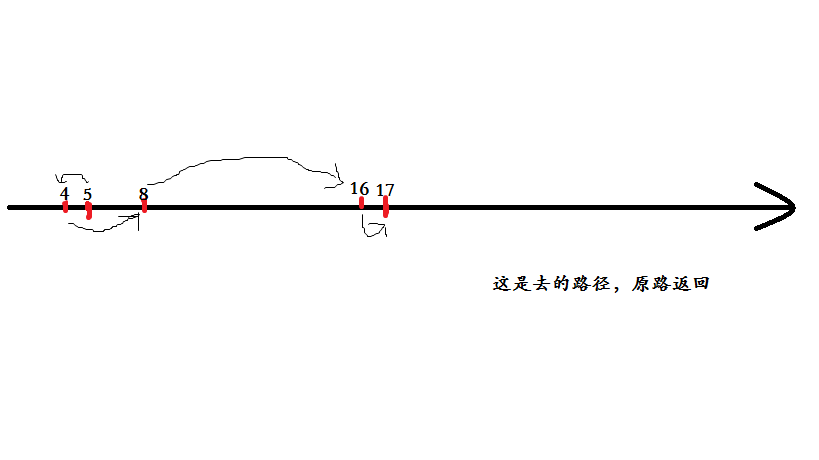# C++广度优先搜索算法之哆啦A梦的时光机

﻿﻿

## Description1. 可以从任意时刻X穿越到X+1或者X-1时刻

2. 可以从任意时刻X穿越到X*2时刻

3. 当X为偶数时，可以从X时刻穿越到X/2时刻

## Sample Input

2
5 17
4 8

## Sample Output

8
2

## 代码

#include<cstdio>
#include<cstring>
const int sb=1e6;
bool mark[sb+1];
int que[sb+1],pre[sb+1],n,k;
int next;
void print(int x)
{
int num=0;
while(pre[x])
{
num++;
x=pre[x];
}
printf("%d\n",num*2);
}
void bfs()
{
if(n==k){printf("0");return ;}
que=n;
mark[n]=true;
{
for(int i=0;i<4;i++)
{
if(next>=0&&next<=sb&&mark[next]!=true)
{
tail++;
que[tail]=next;
mark[next]=true;
if(next==k)
{
print(tail);
break;
}
}
}
}
}
int main()
{
int QAQ;
scanf("%d",&QAQ);
for(int c=0;c<QAQ;c++)
{
scanf("%d%d",&n,&k);
bfs();
memset(mark,false,sizeof(mark));
memset(que,0,sizeof(que));
memset(pre,0,sizeof(pre));
next=0;
}
}## 心得体会﻿﻿